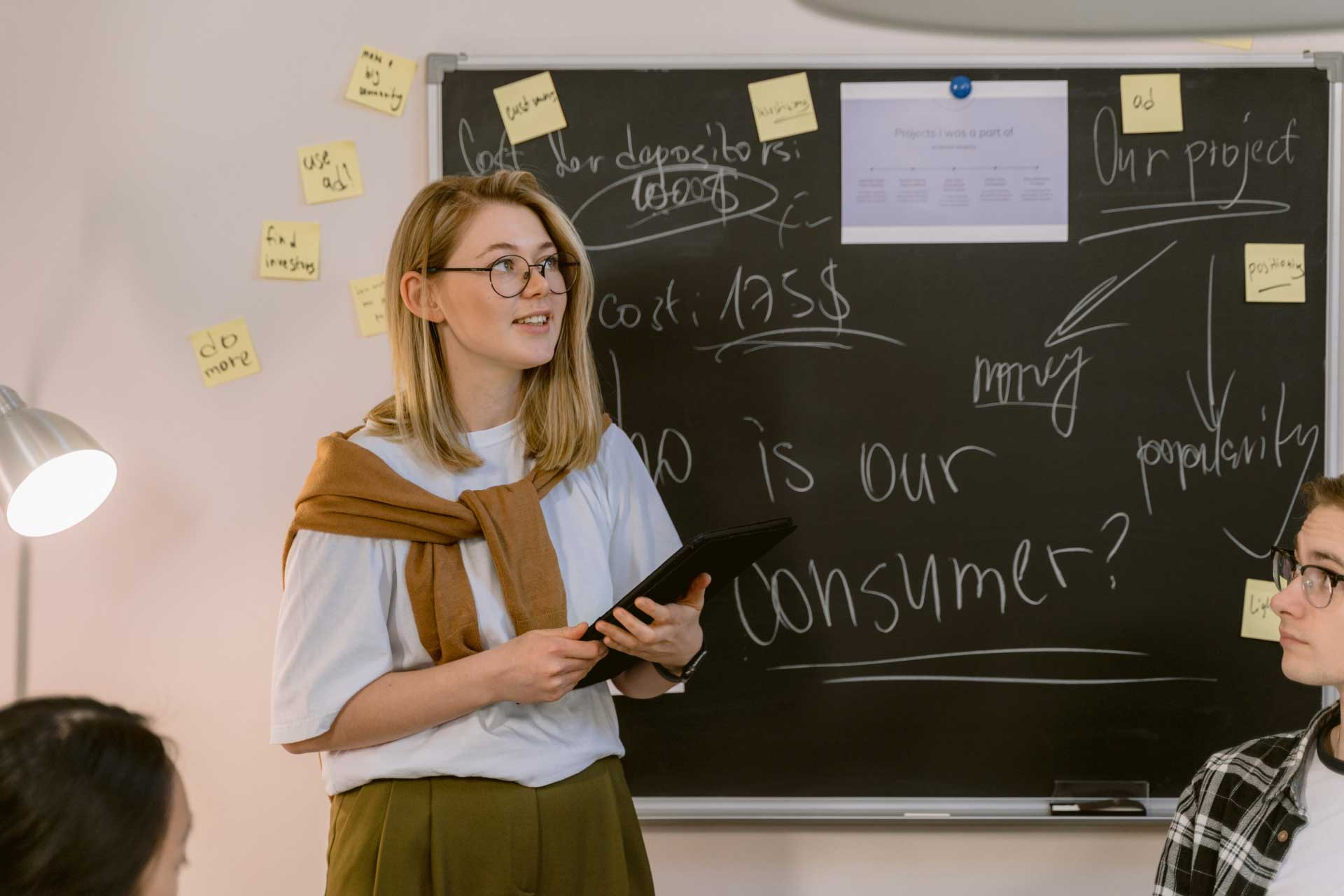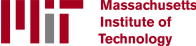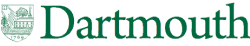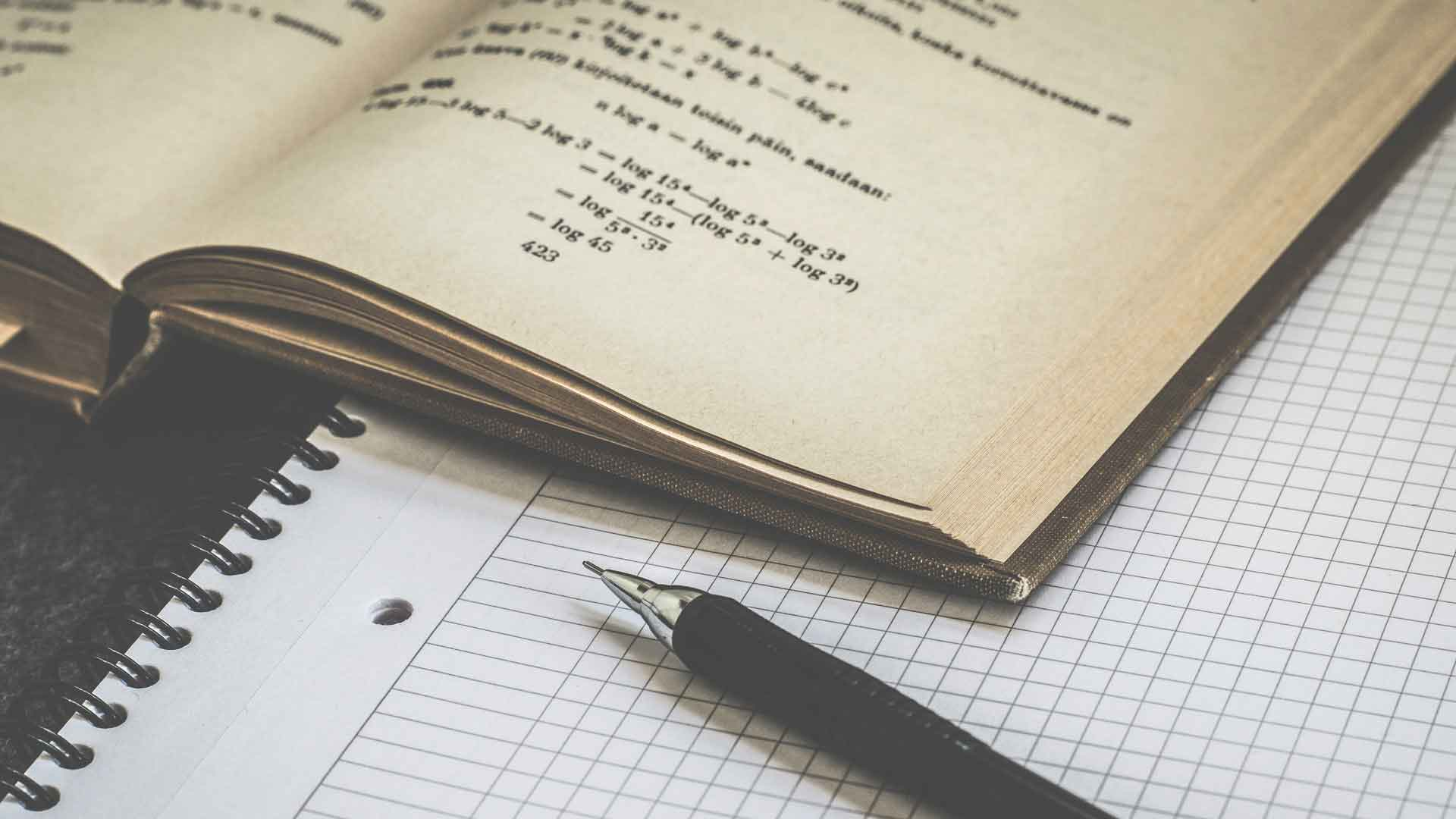# What Are the Best Tips for Teaching Children Mathematics?

There is no underestimating the importance of teaching children mathematics. Children who are able to understand the basic mathematical concepts are much more likely to excel in school. Teaching children mathematics is a progressive process. One should start by teaching the child the basics of addition, followed by subtraction and multiplication, then ending with division, which is often the hardest mathematical concept for a child to learn.

When teaching children mathematics, teaching them addition is the first step in giving them a strong mathematical foundation, which will make learning subtraction, multiplication and division easier. Fortunately, addition is one of the simplest mathematical concepts, and teaching a child the basics of doing simple addition just takes a little time, patience and persistence. The simplest way to start is by teaching the child to add together groups of tangible objects, such as pennies, jelly beans or pebbles. For example, a teacher or parent might place four pennies in one pile and six pennies in another pile, then explain to the child that these two piles represent the addition problem 4 + 6. When the child adds the pennies together and concludes that there are 10 pennies in all, it can be explained that this means that 4 + 6 = 10.

After learning the basics of addition, the next step to teaching kids mathematics is helping them to understand the basic principles of subtraction. As with addition, using tangible objects will make it easier for a child to understand the basic concepts. It will also help them grasp that subtraction is really just addition in reverse. For example, one could start with a pile of 10 jelly beans and allow the child to count them. The teacher can explain to the child that three jelly beans are going to be removed from the pile, which will represent the subtraction problem 10 - 3, and the jelly beans left in the pile will be the answer to the problem. Finally, the child can be allowed to count the seven remaining jelly beans, and the teacher can explain that this means that 10 – 3 = 7.

Multiplication is the next step when teaching children mathematics. When teaching kids multiplication, it is important for the teacher not to progress too fast and to make sure that the children understand the concepts they’ve just been taught before moving on to the next step. A teacher could start by explaining to them that multiplication is really just repeated addition; if there are six piles of pebbles, and there are four pebbles in each pile, that represents the math problem 4 + 4 + 4 + 4 + 4 + 4, which is the same as 6 x 4. After the child understands the concept of repeated addition, he or she can start learning the multiplication tables, also called times tables.Having a thorough understanding of multiplication will make learning basic division a lot easier for a child, but this stage of teaching children mathematics can still be challenging. Once again, using tangible objects can be extremely helpful. For example, a teacher could start with a pile of eight pennies and explain to the child that he or she should divide the pennies into two groups, which will represent the division problem 8 ÷ 2. After the child has split the pennies into two groups, explain that the number of pennies in each separate group is the answer to the problem. When the child counts the pennies in one group, he or she will see that there are four pennies in the group, meaning that 8 ÷ 2 = 4.

At Learner, we offer the world’s best math tutors for your child at the comfort of your own home. Start by taking a short quiz so our Academic Coach can identify your child's learning goals and objectives before custom matching your child with the right tutor.

## Latest Articles### How Can I Score Well on the GED® Math Test?

Passing the General Education Development® (GED®) test gives a person the equivalent of a high school diploma. The math test is one of five sections of the exam. To score well on the GED® math test, you need a sound understanding of the concepts tested in each of the four content areas covered in this section.

Mathematics### What Does a Live Math Tutor Do?

A live math tutor provides individual instruction in areas of math and quantitative sciences. These professionals provide human interaction for students who need face-to-face guidance, and one-on-one teaching to master difficult math topics. Live math tutors offer specialized study guidance according to a particular student’s needs.

Tutoring### What Is Interdisciplinary Mathematics?

Interdisciplinary mathematics is a field of mathematics that merges math expertise with proficiency in another discipline, usually science, business, medicine or engineering. This contemporary training approach to educating math majors caters to employers who need professionals with strong math skills but who have a broader scope of application than traditional mathematics majors.

Mathematics